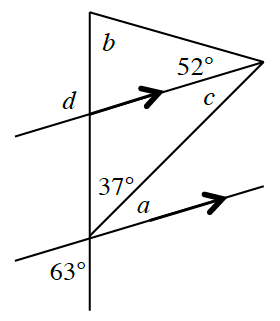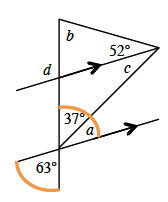### Home > CC4 > Chapter 8 > Lesson 8.2.2 > Problem8-113

8-113.

Use the angle relationships in the diagram below to determine the value of each variable. Name which geometric relationships you used. Homework Help ✎Since the highlighted angles are vertical angles, and vertical angles are equal,
$37º + a = 63º$.

$∠c$ is an alternate interior angle to $∠a$. Recall that alternate interior angles are equal.

Use the Triangle Angle Sum Theorem to calculate $b: 37º + c + 52 + b = 180º$.

To find $m∠d$, notice that it is the exterior angle in the small triangle that contains $∠b$ and $52º$. What relationship do those angles have?